Next: 4. The Complexification of Up: 3. Induction and Integers Previous: 3.4 Summation.   Index

# 3.5 Maximum Function

3.88   Definition (.) Let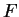be an ordered field, and let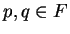. We define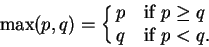Then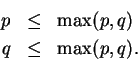3.89   Definition (.) Letbe an ordered field, let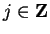and let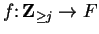be a function. Define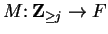by the rules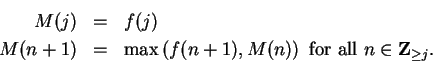Hence, e.g., if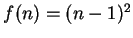,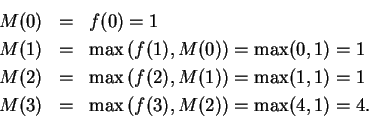We write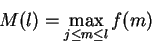where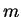is a dummy index, and we think of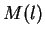as the largest of the numbers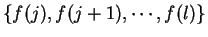. By definition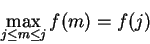and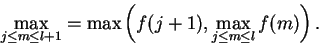3.90   Notation () Let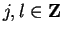with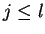. Then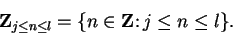3.91   Theorem. Letbe an ordered field, letand letbe a function. Then for all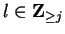,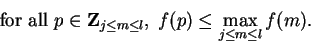(3.92)

Proof: Let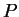be the proposition form on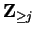such that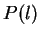is the proposition (3.92). Thensays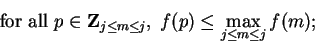i.e.,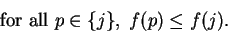Henceis true.

Now for all,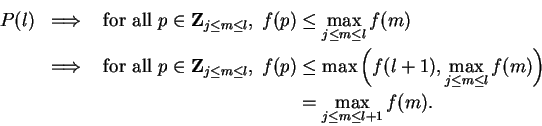We also have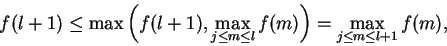so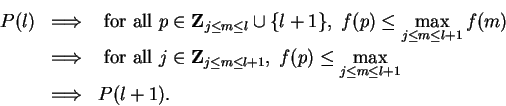By induction,is true for all.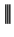3.93   Note. The notation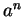for positive integer powers of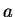was introduced by Descartes in 1637[15, vol 1,p 346]. Both Maple and Mathematica denoteby a^n.

The notation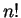for the factorial of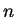was introduced by Christian Kramp in 1808[15, vol 2, p 66].

The use of the Greek letter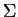to denote sums was introduced by Euler in 1755[15, vol 2,p 61]. Euler writes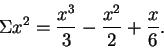The use of limits on sums was introduced by Augustin Cauchy(1789-1857). Cauchy used the notationto denote what we would write as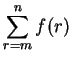[15, vol 2, p 61].

In Maple, the value ofis denoted by sum(f(i),i=1..n). In Mathematica it is denoted by Sum[f[i],{i,1,n}]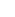.Next: 4. The Complexification of Up: 3. Induction and Integers Previous: 3.4 Summation.   Index